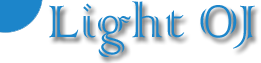# [Solved] 1225 – Palindromic Numbers (II) of LightOJ### [Solved] 1225 – Palindromic Numbers (II) of LightOJ

#### Main LightOJ Problem is here

A palindromic number or numeral palindrome is a
‘symmetrical’ number like 16461, that remains the same when its digits are
reversed. In this problem you will be given an integer, you have to say whether
the number is a palindromic number or not.

# Input

Input starts with an integer T (≤ 20000),
denoting the number of test cases.

Each case starts with a line containing an integer n (0 ≤
n < 109)
.

# Output

For each case, print the case number and ‘Yes’ if n
is palindromic, otherwise print ‘No’.

# Output for Sample Input

5

1

21

16161

523125

0

Case 1: Yes

Case 2: No

Case 3: Yes

Case 4: No

Case 5: Yes

#### Full Source Code Using C Plus Plus

Code
1. #include<iostream>
2. #include<cstring>
3. #include<stdio.h>
4. using namespace std;
5. int main()
6. {
7.     int t,i,j,k,flag=1;
8.     char n;
9.     cin>>t;
10.     for(i=1;i<=t;i++)
11.     {
12.         flag=1;
13.         scanf(“%s”,n);
14.         for(j=0,k=strlen(n)1;k>=j;j++)
15.         {
16.             if(n[j]!=n[kj])
17.             {
18.                 flag=0;
19.                 break;
20.             }
21.         }
22.         if(flag==1) cout<<“Case “<<i<<“: Yes\n;
23.         else cout<<“Case “<<i<<“: No\n;
24.     }
25.     return 0;
26. }
27.